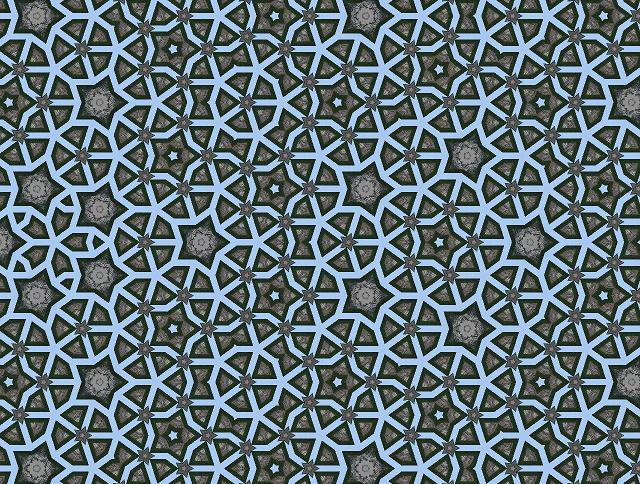News: Visit the official fractalforums.com Youtube Channel## The All New FractalForums is now in Public Beta Testing! Visit FractalForums.org and check it out!

pentagonal caleidoscopePrevious Image | Next Image
Description: The product of my attempt to KIFS-ify () Penrose tiling. It's aperiodic but not a Penrose tiling.
The evaldraw script:
Code:
static a=-sin(4*pi/5),b=cos(4*pi/5),c=sin(4*pi/5);
static phi=0.5*(1+sqrt(5));
(x,y,&r,&g,&b){
n=10;k=1/phi^n;
x*=k;y*=k;
x+=0.5;
for(i=0;i<n;i++) iter(x,y);

pic(x*256+mousx,mousy-y*256,r,g,b)
}
iter(&x,&y){

t=2*min(0,a*x+b*y+c);
x-=t*a; y-=t*b;
y=abs(y);
x*=phi;y*=phi;
x=phi-x;
t=2*min(0,a*x+b*y+c);
x-=t*a; y-=t*b;
y=abs(y);
}

enjoy
Stats:
Total Favorities: 0 View Who Favorited
Filesize: 139.25kB
Height: 484 Width: 640
Discussion Topic: View Topic
Keywords: Kaleidoscope aperiodic tiling Penrose evaldraw
Posted by: knightyDecember 02, 2012, 08:52:05 PM

Rating:by 2 members.